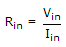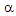# Electronics - Bipolar Junction Transistors (BJT)

### Exercise :: Bipolar Junction Transistors (BJT) - Filling the Blanks

1.

The input resistance of the base of an emitter-follower is usually _________.

 A. very low B. very high C. shorted to ground D. open

Answer: Option B

Explanation:

No answer description available for this question. Let us discuss.

2.

The phase difference between the input and output ac voltage signals of a common-emitter amplifier is __________.

 A. 0º B. 90º C. 180º D. 360º

Answer: Option C

Explanation:

No answer description available for this question. Let us discuss.

3.

Base bias provides _________.

 A. a very stable Q point B. a very unstable Q point C. no current gain D. zero current in the base and collector circuits

Answer: Option B

Explanation:

No answer description available for this question. Let us discuss.

4.

VCE approximately equals ________ when a transistor switch is cut off.

 A. VCC B. VB C. 0.2 V D. 0.7 V

Answer: Option A

Explanation:

No answer description available for this question. Let us discuss.

5.

The input resistance, Rin of a common-collector amplifier, consists of ____________.

 A. Rin = RE × AC Current Gain B.C. Rin = RE ×D. none of the above

Answer: Option A

Explanation:

No answer description available for this question. Let us discuss.

#### Current Affairs 2021

Interview Questions and Answers